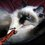# Kinematic equations derived from Calculus.

First important relation:

Consider the graph of a function $\displaystyle f(t)$ which takes as an input time and outputs velocity at that particular time. From calculus we know that the area under the graph of this function between time $\displaystyle t_i$ and $\displaystyle t_f$ is: $\Delta_x=x_f-x_i=\int_{t_i}^{t_f}f(t)\,\mathrm dt.$ The same thing can be performed with a function $\displaystyle g(t)$ that outputs for each time, the acceleration at that time. And so the area under the graph of this function between time $\displaystyle t_i$ and $\displaystyle t_f$ is: $\Delta_{v}=v_{xf}-v_{xi}=\int_{t_i}^{t_f}g(t)\,\mathrm dt.$

Kinematics!

Acceleration is defined as $a_x=\dfrac{\mathrm d v_x}{\mathrm d t}.$ We can rearrange it to get $\displaystyle \mathrm dv_x=a_x\,\mathrm dt.$ If we integrate both sides and consider our first relation, and letting $\displaystyle t_i=0$ and $\displaystyle t_f=t$, we get that: $v_{xf}-v_{xi}=\int_0^t a_x\,\mathrm dt.$ And if acceleration is constant, and using the fundamental theorem of calculus, we get that: $v_{xf}-v_{xi}=\int_0^t a_x\,\mathrm dt=a_x(t-0)=a_xt.$ If we add $\displaystyle v_{xi}$ to both sides we obtain: $\color{grey}{\boxed{\color{#FFFFFF}{\overline{\color{#FFFFFF}{\underline{\color{#333333}{ v_{xf}=v_{xi}+a_x\,t. }}}}}}}$ This relation will let us find the final velocity at time $\displaystyle t$ if we know the initial velocity $\displaystyle v_{xi}$ and the (constant) acceleration $\displaystyle a_x$.

In the same spirit, we can derive another kinematics equation by recalling that velocity is: $v_{x}=\dfrac{\mathrm dx}{\mathrm dt}.$ Rearranging we get $\displaystyle \mathrm dx=v_{x}\,\mathrm dt.$ If we integrate both sides and using our very first relation we get that: $\Delta_x=x_f-x_i=\int_{0}^t v_{x}\,\mathrm dt.$ And since $\displaystyle v_{x}=v_{xf}=v_{xi}+a_xt$ we can rewrite our definite integral as \eqalign{ x_f-x_i&=\int_{0}^t v_{x}\,\mathrm dt \\ &=\int_0^t\left(v_{xi}+a_xt\right)\mathrm dt \\ &= \int_0^tv_{xi}\,\mathrm dt+a_x\int_0^t t\,\mathrm dt \\ &=v_{xi}t+a_x\dfrac{t^2}{2} \\ &=v_{xi}+\tfrac12a_xt^2.} Adding $\displaystyle x_i$ to both sides we get: $\color{grey}{\boxed{\color{#FFFFFF}{\overline{\color{#FFFFFF}{\underline{\color{#333333}{ x_{f}=x_i+v_{xi}t+\tfrac12a_x\,t^2. }}}}}}}$ This relation let us determine the final position $\displaystyle x_f$ at time $\displaystyle t$ if we know the initial position $\displaystyle x_i$ with the initial velocity $\displaystyle x_i$ and if the acceleration $\displaystyle a_x$ is constant and well-known.

I hope this helps. Best wishes, $\displaystyle \cal H$akim.Note by حكيم الفيلسوف الضائع
7 years, 1 month ago

This discussion board is a place to discuss our Daily Challenges and the math and science related to those challenges. Explanations are more than just a solution — they should explain the steps and thinking strategies that you used to obtain the solution. Comments should further the discussion of math and science.

When posting on Brilliant:

• Use the emojis to react to an explanation, whether you're congratulating a job well done , or just really confused .
• Ask specific questions about the challenge or the steps in somebody's explanation. Well-posed questions can add a lot to the discussion, but posting "I don't understand!" doesn't help anyone.
• Try to contribute something new to the discussion, whether it is an extension, generalization or other idea related to the challenge.

MarkdownAppears as
*italics* or _italics_ italics
**bold** or __bold__ bold
- bulleted- list
• bulleted
• list
1. numbered2. list
1. numbered
2. list
Note: you must add a full line of space before and after lists for them to show up correctly
paragraph 1paragraph 2

paragraph 1

paragraph 2

[example link](https://brilliant.org)example link
> This is a quote
This is a quote
    # I indented these lines
# 4 spaces, and now they show
# up as a code block.

print "hello world"
# I indented these lines
# 4 spaces, and now they show
# up as a code block.

print "hello world"
MathAppears as
Remember to wrap math in $$ ... $$ or $ ... $ to ensure proper formatting.
2 \times 3 $2 \times 3$
2^{34} $2^{34}$
a_{i-1} $a_{i-1}$
\frac{2}{3} $\frac{2}{3}$
\sqrt{2} $\sqrt{2}$
\sum_{i=1}^3 $\sum_{i=1}^3$
\sin \theta $\sin \theta$
\boxed{123} $\boxed{123}$

Sort by:

شكرا ولد لبلاد

- 6 years, 10 months ago

hhh :-D

- 6 years, 10 months ago

Nice explanation

- 7 years, 1 month ago

Glad you liked it! $\overset{\cdot\cdot}\smile$

Wow excellent thanks I didn't know this

- 7 years, 1 month ago

Glad you liked it! $\overset{\cdot\cdot}\smile$

Vf^2=vo^2+2as? Put that in your discussion.

Bonus: try incorporating the chain rule.

- 2 years, 8 months ago

We can even derive Torricelli's Equations, such as $v_f^{2}-v_i^{2}=2as$.

- 7 years, 1 month ago

Yea I agree by the way what grade are you in

- 7 years, 1 month ago

11th

- 7 years, 1 month ago

this is good...basic of kinematics ..thanks for recalling..

- 7 years ago

equation of motion already explained in INDIAN NCERT book by this method but not in perfect way .here this one is explained in perfect way.

- 7 years ago

No meaning of level 3. These derivations are known to every 9th standard student.

- 6 years, 11 months ago